1. Price inflationThis year the average wage grew by 4.5%. Prices rose by 2%. How much did the real wage increase in the given year?
2. ParkingAt a building parking, 245 spaces are for cars, 56 are for vans and the rest are for buses. If 14% of all parking spaces are for buses, how many parking spaces are there in the building? How many spaces are for buses?
3. Octane value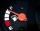I loaded 10L 95 octane gasoline and 5L 100 octane gasoline. What is the resulting octane value of the gasoline in the tank?
4. Sale discountThe product was discounted so that eight products at a new price cost just as five products at an old price. How many percents is the new price lower than the old price?
5. A truckA truck departs from a distribution center. From there, it goes 20km west, 30km north and 10km west and reaches a shop. How can the truck reach back to the distribution center from the shop (what is the shortest path)?
6. 7 digit numberIf 3c54d10 is divisible by 330, what is the sum of c and d?
7. Wiring 2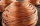Willie cut a piece of wire that was 3/8 of the total length of the wire. He cut another piece that was 9m long. The two pieces together were one half of the total length of the wire. How long was the wire before he cut it?
8. Electric input powerSolve problems related to electric power: a) U = 120 V, I = 0.5 A, P =? b) P = 200 W, U = 230 V, I =? c) I = 5 A, P = 2200W, U =?
9. TransformerSolve the textbook problems - transformer: a) N1 = 40, N2 = 80, U2 = 80 V, U1 =? b) N1 = 400, U1 = 200 V, U2 = 50 V, N2 =?
10. Two pumpsPump A takes twice as long to fill a tank than B . Together they take 2 hours to fill the tank. How long does each one take to fill the tank by itself?
11. Cone 15The radius of the base of a right circular cone is 14 inches and it's height 18 inches. What is the slant height?
12. Butter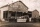At the Orville redenbocher popcorn factory there is a tank of artificial butter substitute which is 55 feet tall and 18 feet in diameter. How many gallons of artificial butter substitute can the tank contain?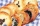Montealegre family has a catering business. In one birthday party, they charged their costumer 11,767.50 for a party of 90 persons and a service charge of 2,500.50. How much was the average cost per person? Please show your solution.
14. The largerThe larger of two numbers is nine more than four times the smaller number. The sum of the two numbers is fifty-nine. Find the two numbers.
15. Supermarket 2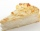A supermarket had a buko pie sale. In the morning 2/3 of the pies were sold and in the afternoon 1/6 of the pies were sold. If 150 pies were left, how many pies had been sold? Show your solution.
16. Last digitWhat is the last number of 2016 power of 2017
17. Peaches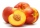There are 20 peaches in the pocket. 3 peaches are rotten. What is the probability that one of the randomly picked two peaches will be just one rotten?
18. Three sidesSide b is 2 cm longer than side c, side a is 9 cm shorter than side b. The triangle circumference is 40 cm. Find the length of sides a, b, c . .. .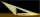A meter pole perpendicular to the ground throws a shadow of 40 cm long, the house throws a shadow 6 meters long. What is the height of the house?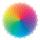Dana confuses sweater and wool has a choice of seven colors. In how many ways she can choose from three colors to the sleeves?